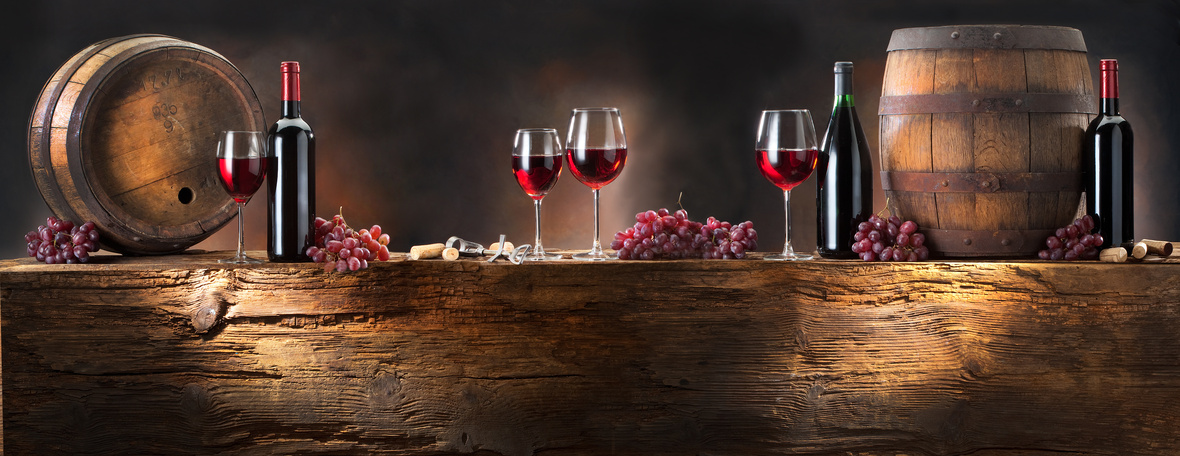# Birthday Month Recap & TV Appearances Galore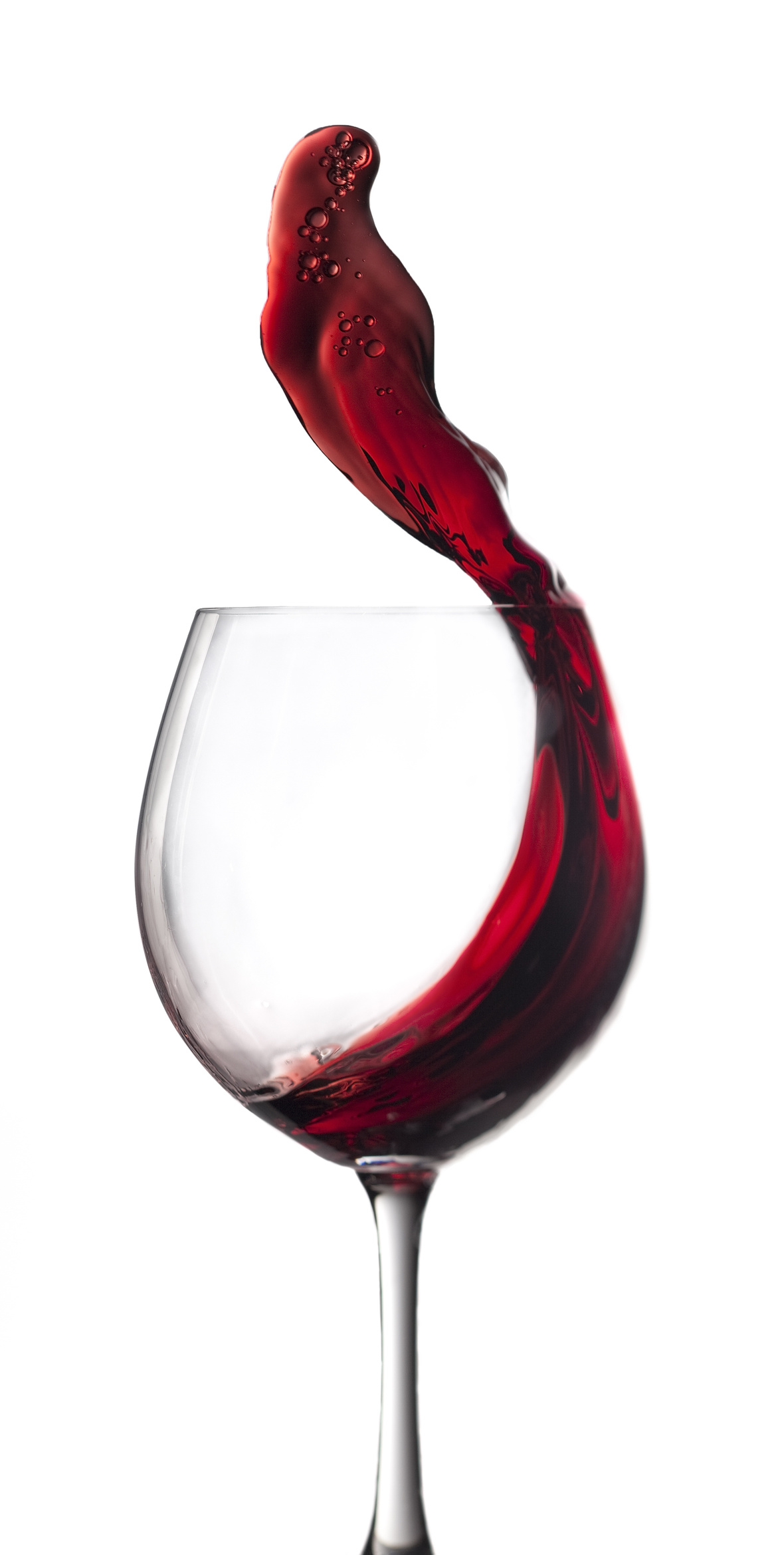Hi Wine Lover,

The Shall We Wine team is excited to share our monthly newsletter. Our goal is to introduce you to our favorite wines, winemakers, varietals, wine regions and places to buy and drink wine.

Cheers,

The Shall We Wine Team

 /* styles */
 table div table+table+table+table+table div table{width:100%;padding:0}table div table+table+table+table+table div table img{width:96.23%;padding:0;float:none}table div table+table+table+table+table div table td{width:100%;padding:0 1.88% 18px}/* styles */## SISTER CIRCLE LIVE

 /* styles */ If there's any advice at all to give, it's to grind towards your vision. Birthday month was filled with many blessings - none of which would have been possible without the daily grind and a little elbow grease! I returned to the Sister Circle Live set for a special wine & food pairing segment with Blu Lagoon Atlanta. Icing on top? Being set buddies with the amazing Brian McKnight!Missed the segment?

For more info on the wines featured on SISTER CIRCLE LIVE, check out the "DRINK THIS" section below in this newsletter!

 table div table+table+table+table+table+table+table+table+table+table div table{width:100%;padding:0}table div table+table+table+table+table+table+table+table+table+table div table img{width:96.23%;padding:0;float:none}table div table+table+table+table+table+table+table+table+table+table div table td{width:100%;padding:0 1.88% 18px}/* styles */## BÂTTONNAGE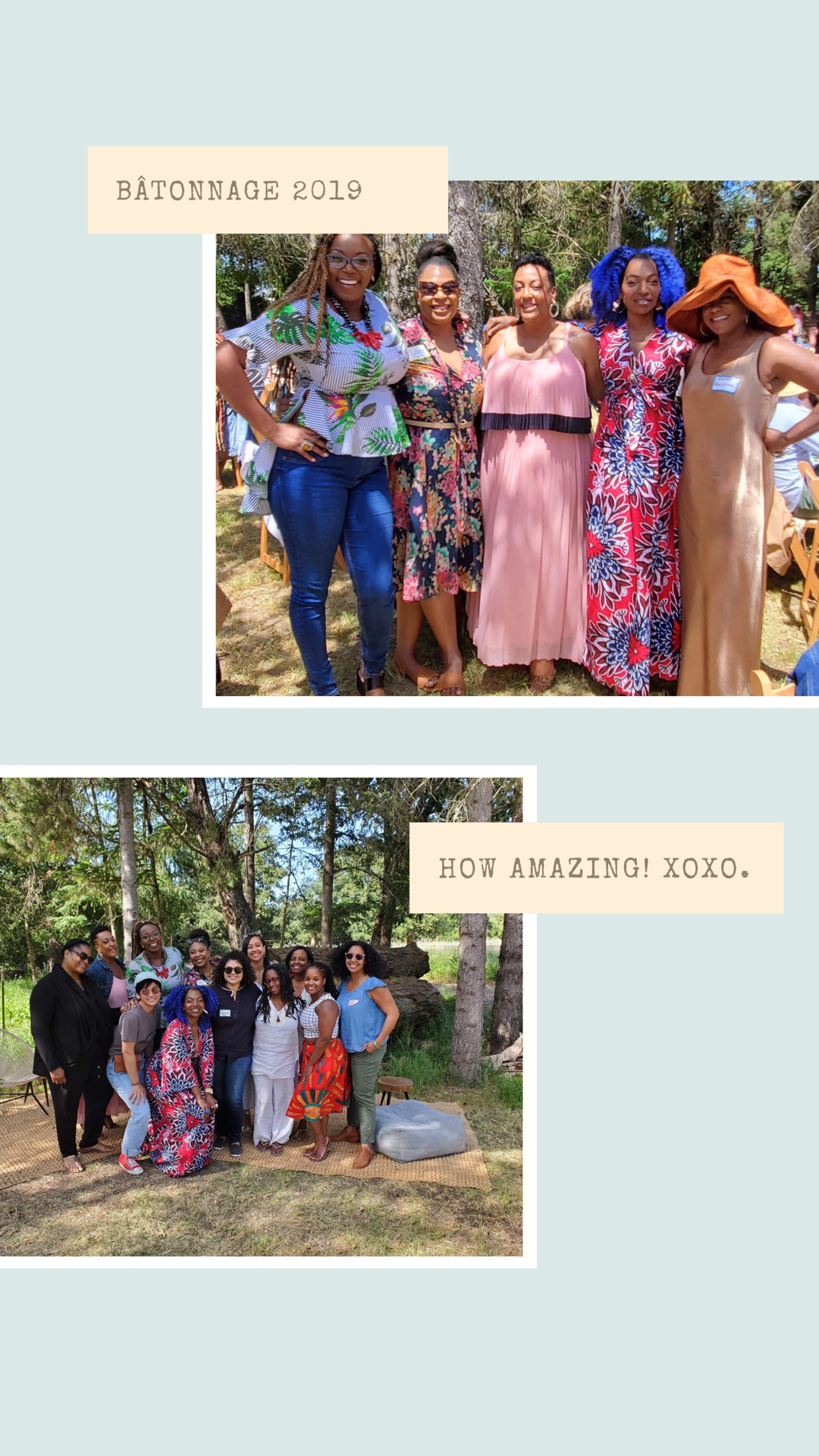Bâtonnage Forum takes its name from the French term meaning "lees stirring". True to the origin of its name, the 2019 forum provided a platform for those “who have traditionally been overlooked or spoken over” in the beverage industry to stir things up.

Y’all, the conversation was raw, heated and operative. The one day event’s agenda included three panel discussions, lunch and a post event wrap up happy hour. What I valued most about this conference was the panelists' commitment to taking off the masque. The panel topics (listed below) were fresh and allowed for candid dialogue.

Panel 1 - Departing Dysfunction
Panel 2 - Pathways to Inclusion: But How, Really? Panel 3 - Do You Sell Sex?

Grateful for the opportunity to attend this sold out forum in Napa, CA and be a part of the conversation about us ladies in various facets of the wine industry!

 table div table+table+table+table+table+table+table+table+table+table+table+table+table div table{width:100%;padding:0}table div table+table+table+table+table+table+table+table+table+table+table+table+table div table img{width:96.23%;padding:0;float:none}table div table+table+table+table+table+table+table+table+table+table+table+table+table div table td{width:100%;padding:0 1.88% 18px}/* styles */## LET'S TALK LIVE & GREAT DAY WASHINGTON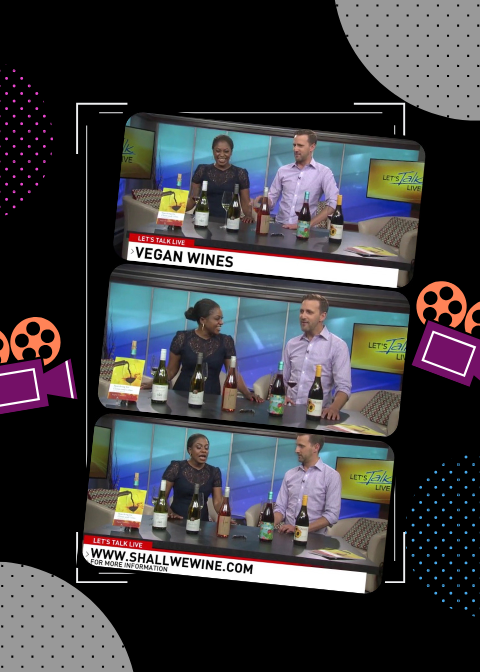Washington, D.C. what a time! Thank you for wine-ing with me, I had a blast.

On Let's Talk Live we talked about Vegan Wine. Hope everyone learned a thing or two about what vegan wine is and some great tasting options to look out for!

Missed the segment?

For more info on the wines featured on LET'S TALK LIVE, check out the "DRINK THIS" section below in this newsletter!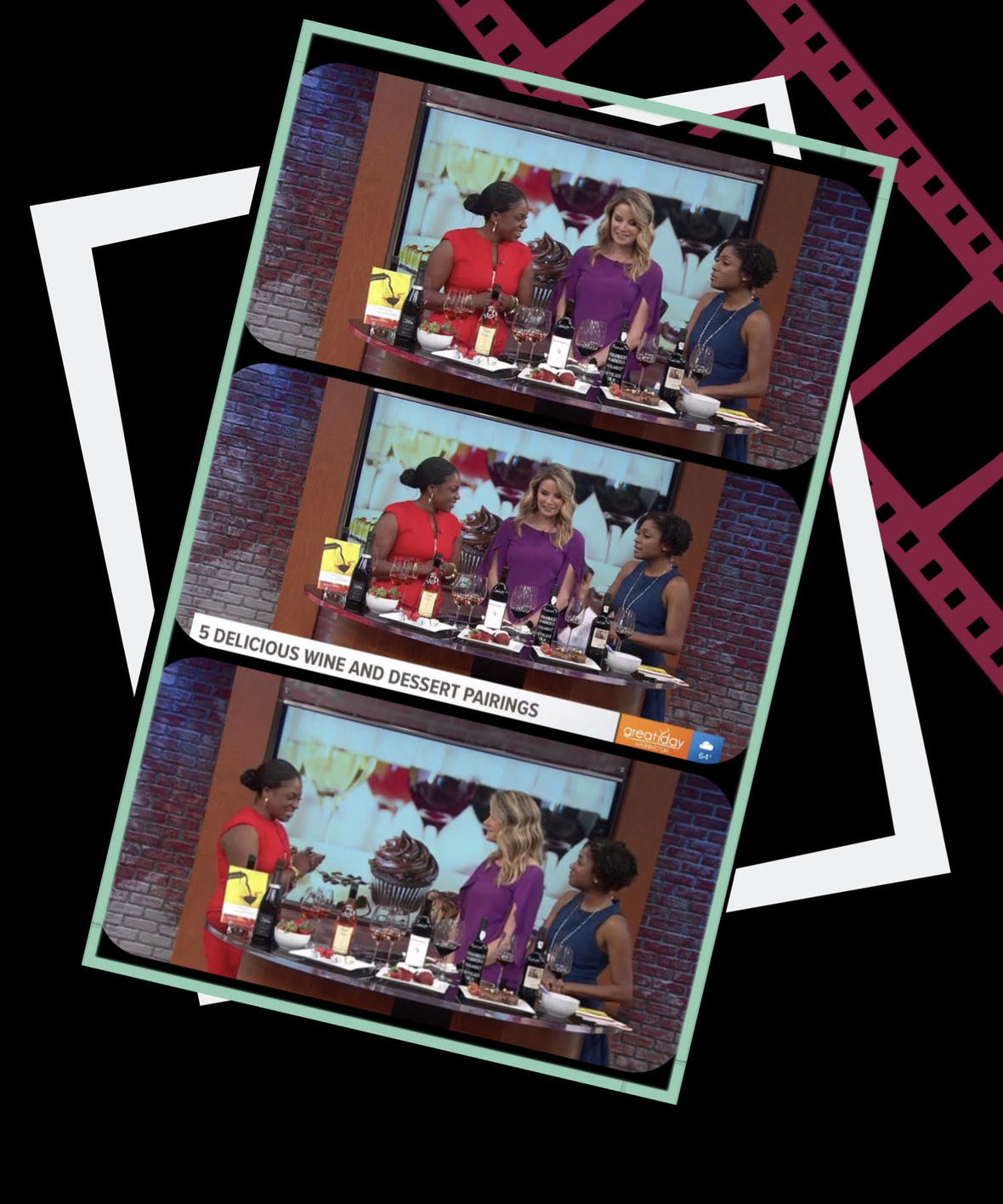On Great Day Washington we talked wine & dessert pairings. Hope everyone learned a thing or two about pairing spring wine with dessert!

Special shoutouts to Buttercream Bakeshop and Riedel USA for dessert and glassware!

Missed the segment?

For more info on the wines featured on GREAT DAY WASHINGTON, check out the "DRINK THIS" section below in this newsletter!⠀⠀⠀⠀⠀⠀⠀⠀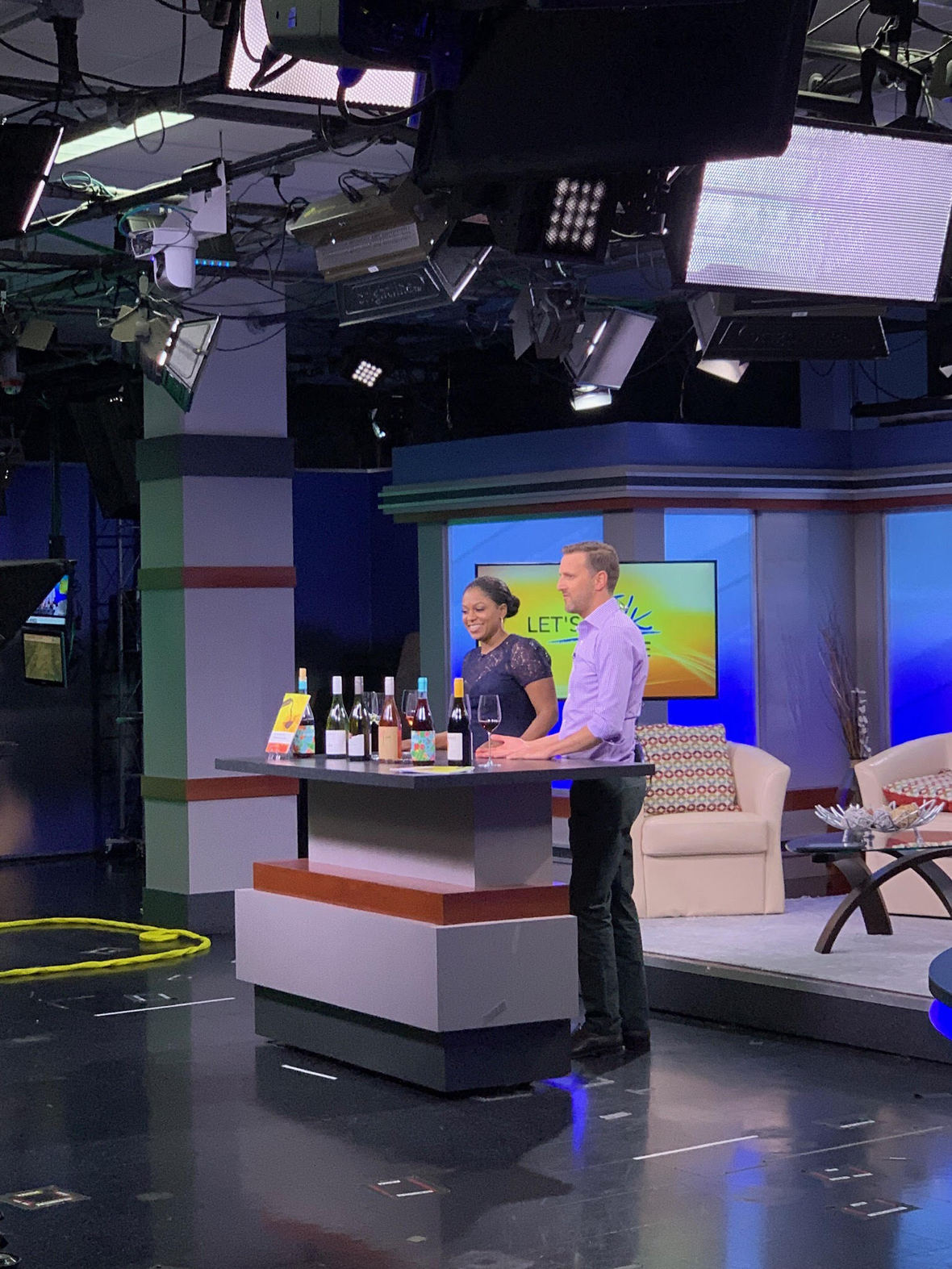ON SET - LET'S TALK LIVE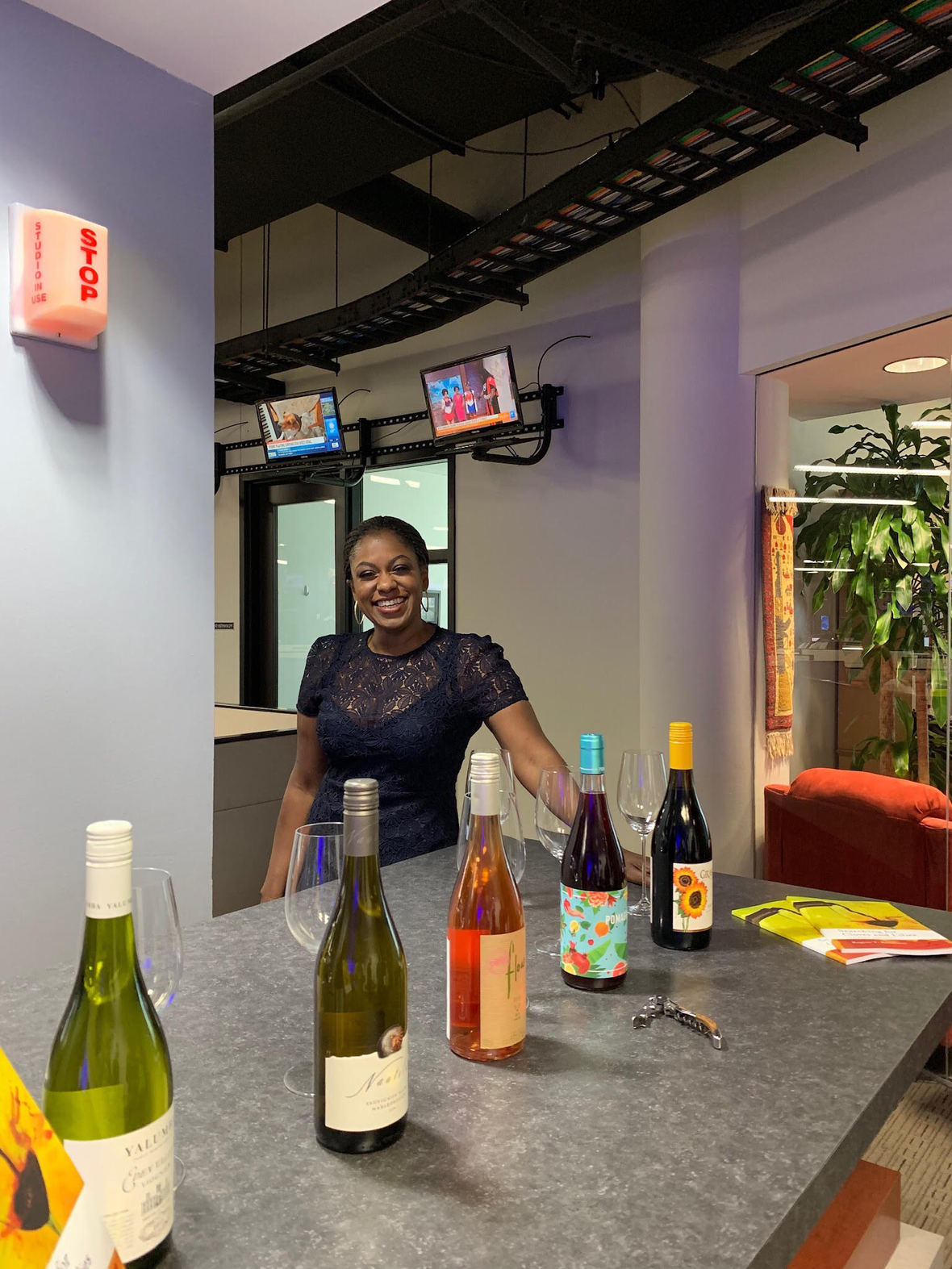BEHIND THE SCENES - LET'S TALK LIVE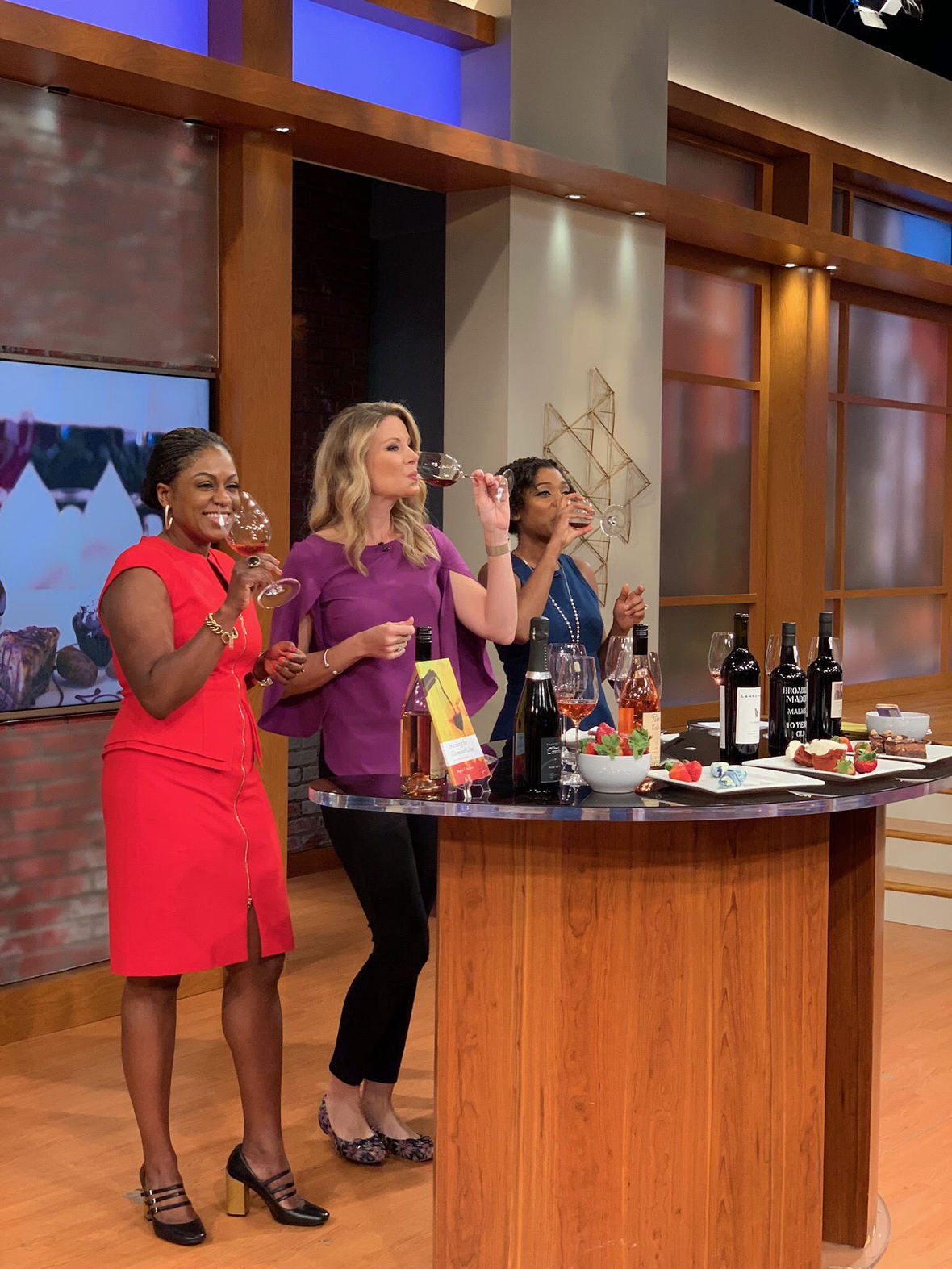TASTE TEST - GREAT DAY WASH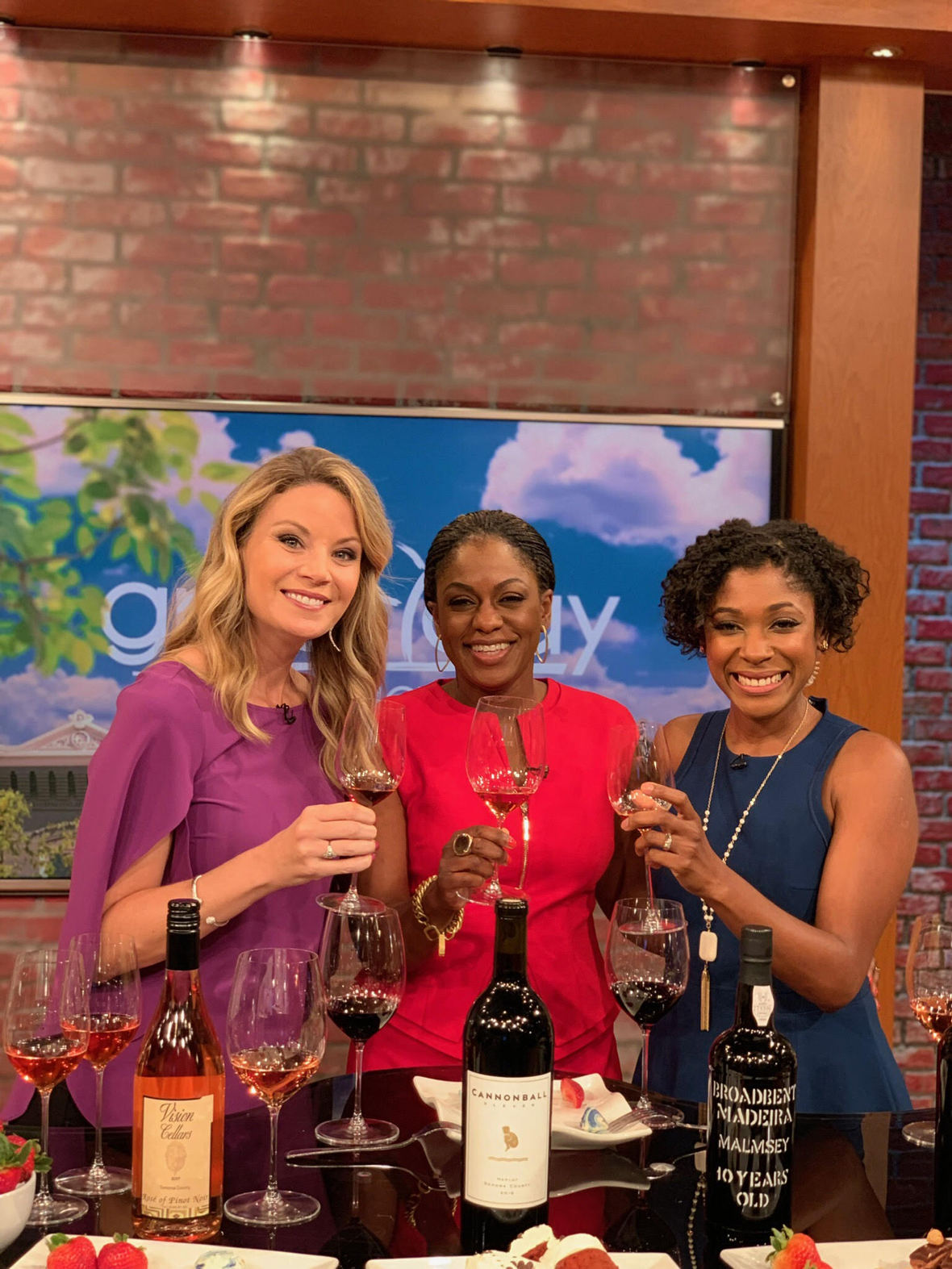CHEERS - GREAT DAY WASH
 table div table+table+table+table+table+table+table+table+table+table+table+table+table+table+table+table+table+table+table div table{width:100%;padding:0}table div table+table+table+table+table+table+table+table+table+table+table+table+table+table+table+table+table+table+table div table img{width:96.23%;padding:0;float:none}table div table+table+table+table+table+table+table+table+table+table+table+table+table+table+table+table+table+table+table div table td{width:100%;padding:0 1.88% 18px}/* styles *//* styles */ Reader inspiration in Santa Rosa: This is #AuthorLife! Participated in an author poetry reading & wine pairing event at Astro Motel, sharing my book Searching for Cloves & Lilies: The Wine Edition to a crowd of new readers! Amazing.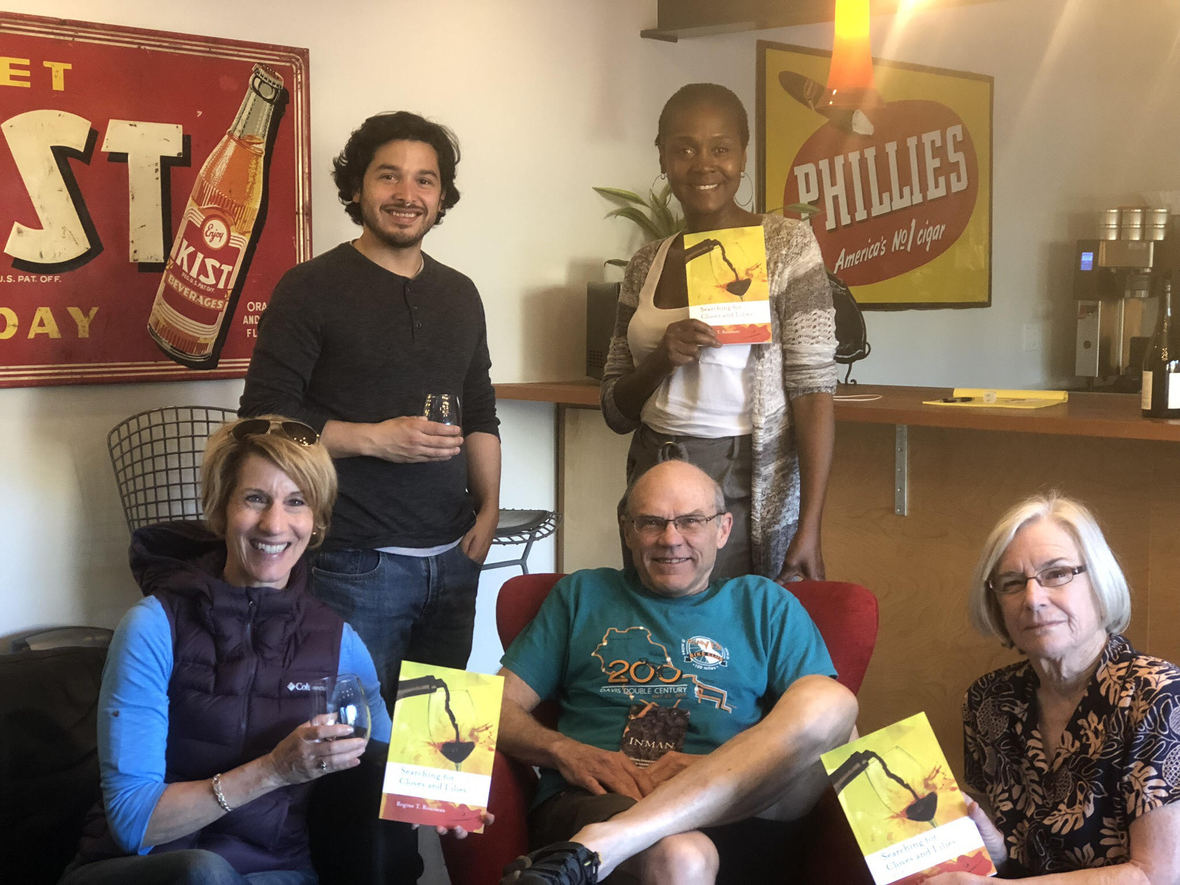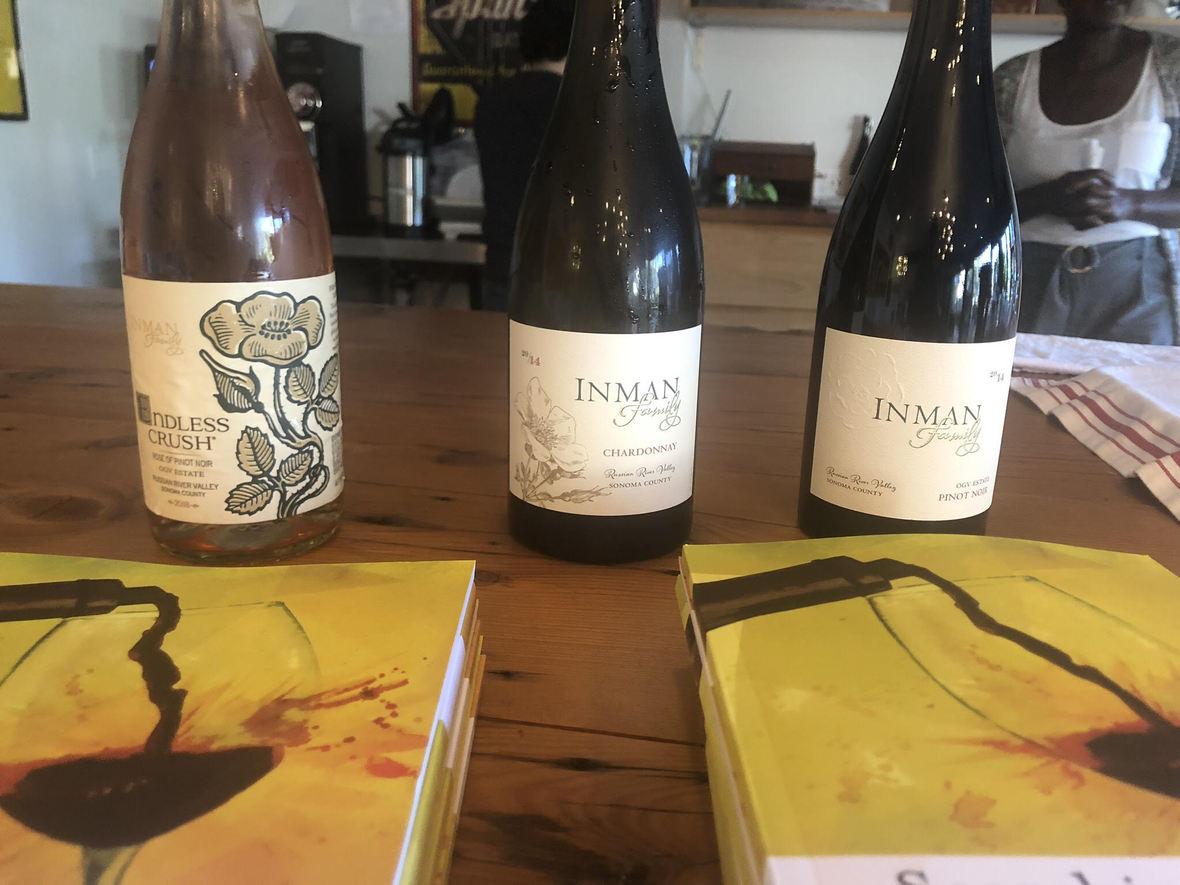# Let's keep inspiring new readers in 2019!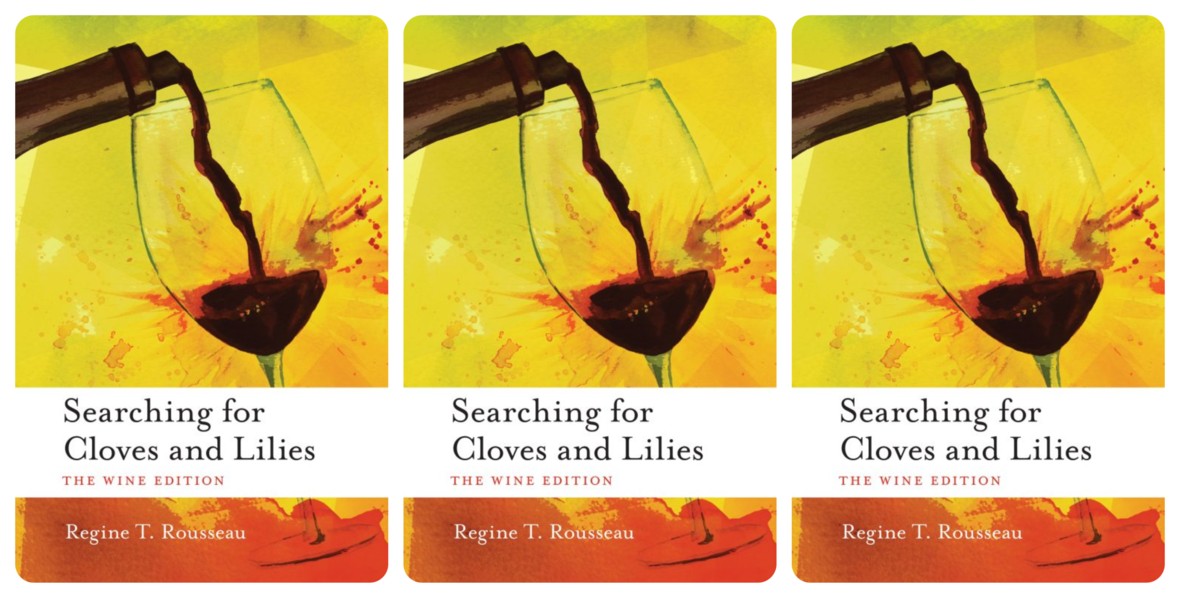table div table+table+table+table+table+table+table+table+table+table+table+table+table+table+table+table+table+table+table+table+table+table+table+table+table+table div table{width:100%;padding:0}table div table+table+table+table+table+table+table+table+table+table+table+table+table+table+table+table+table+table+table+table+table+table+table+table+table+table div table img{width:96.23%;padding:0;float:none}table div table+table+table+table+table+table+table+table+table+table+table+table+table+table+table+table+table+table+table+table+table+table+table+table+table+table div table td{width:100%;padding:0 1.88% 18px}/* styles *//* styles */

## WORDS & WINE: CITY WINERY

 /* styles */ Thank you all who attended the sold out second installment of Words & Wine at City Winery Chicago! It was a fabulous poetry, music and wine pairing event, featuring sounds by The Lab3l.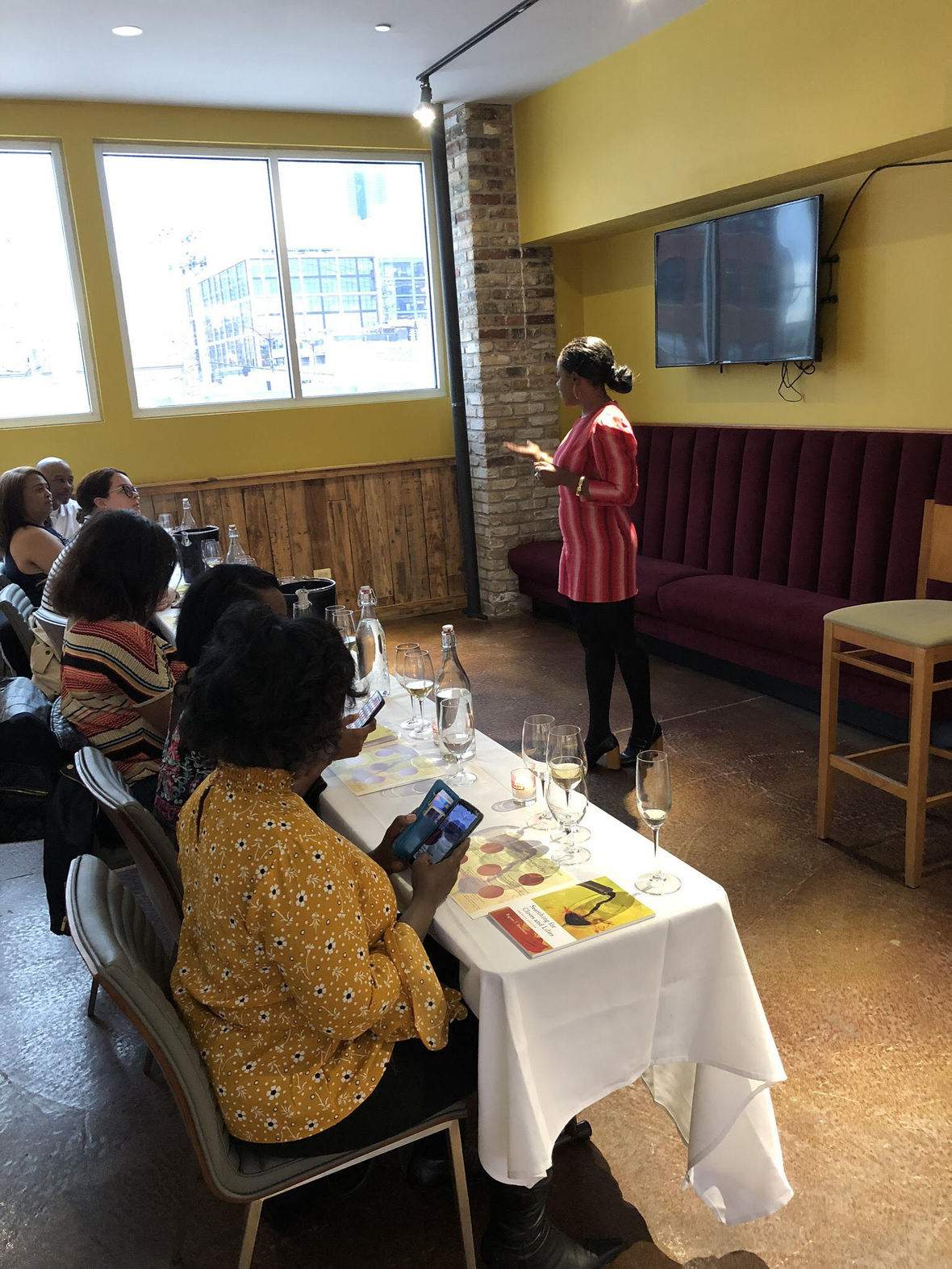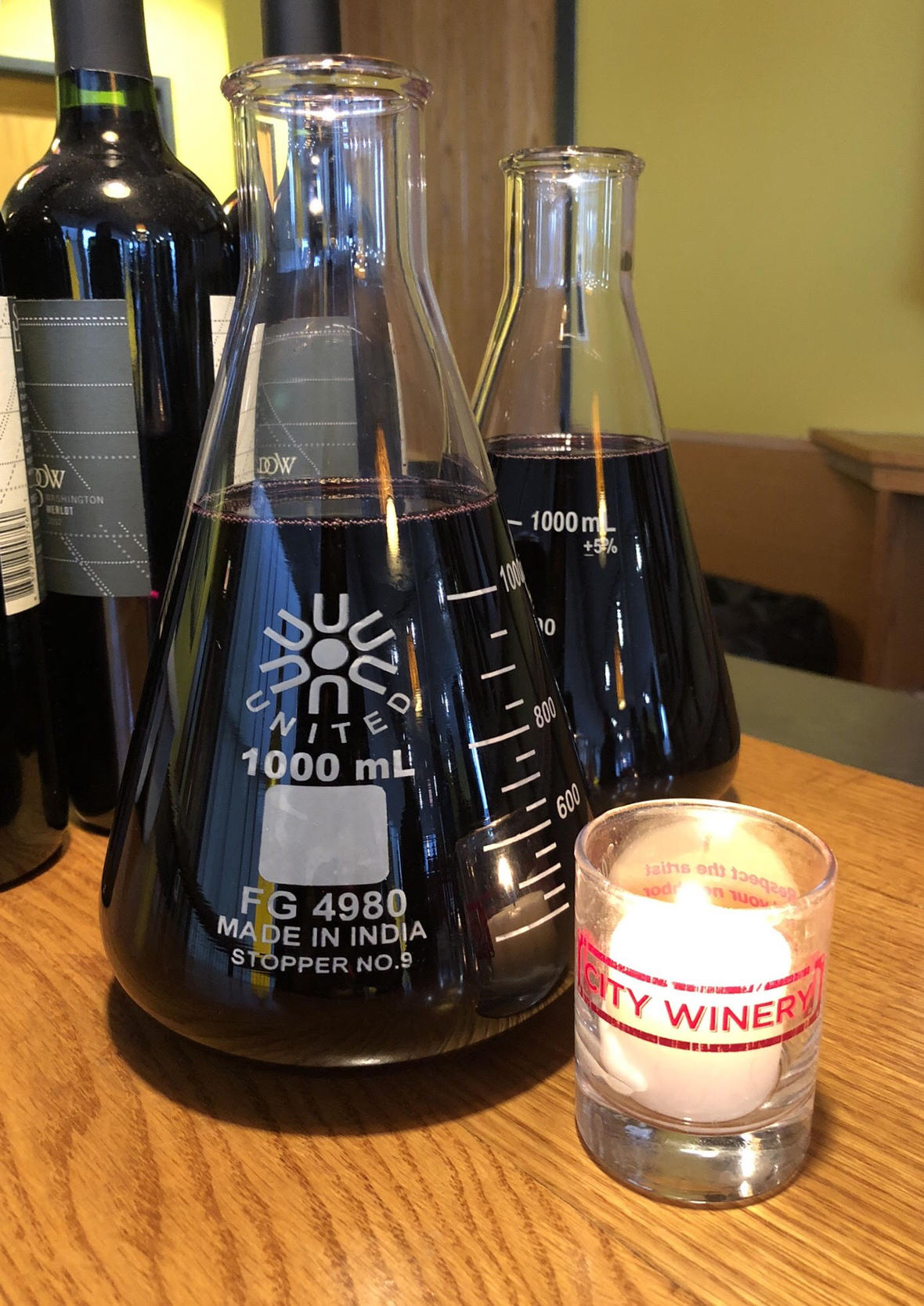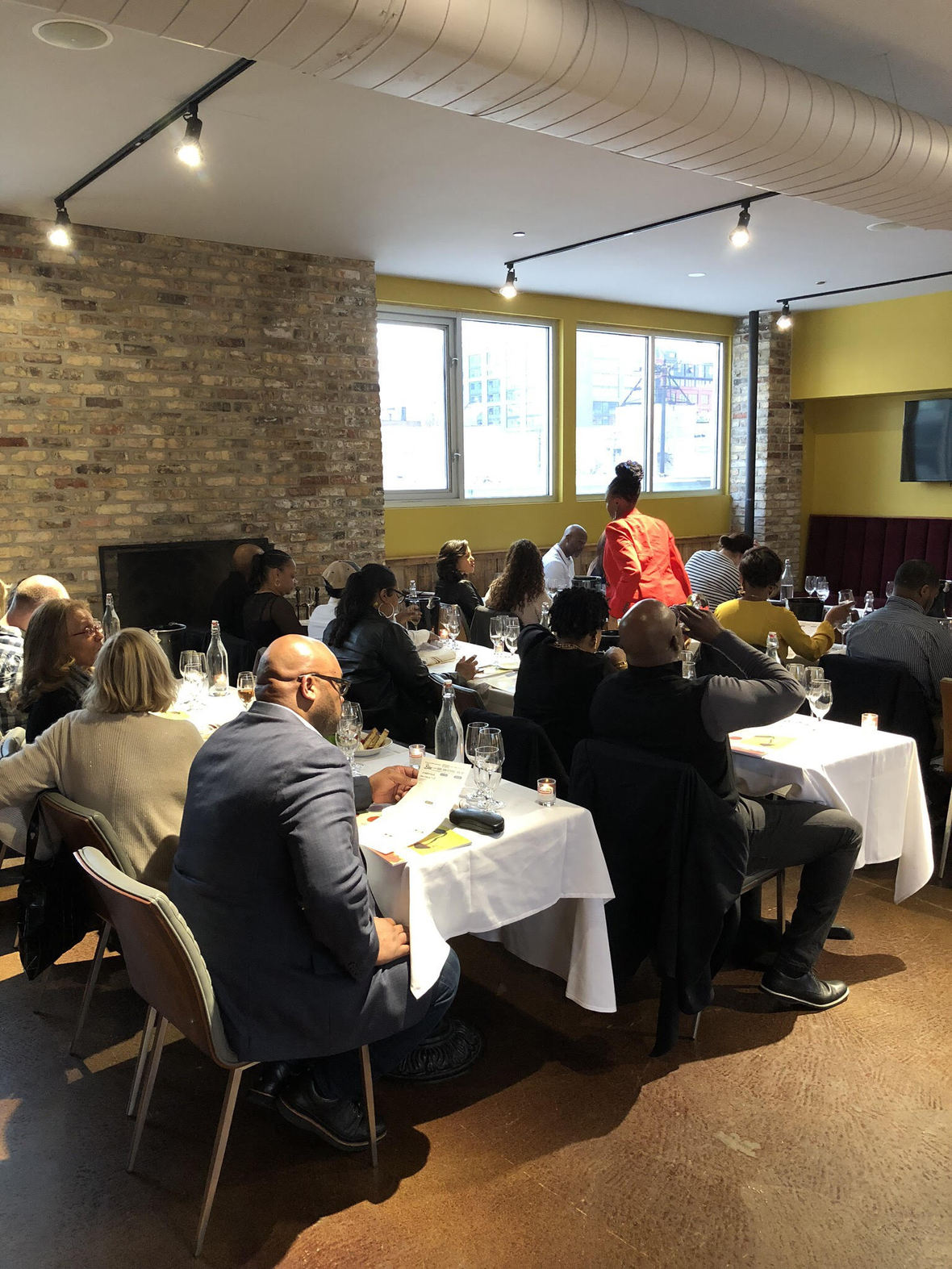/* styles */ As both a sommelier and poet, I see wine elevated by words and vice versa. On the surface, wine and poetry can seem unrelated. Searching for Cloves & Lilies: The Wine Edition is one way of showing the congruence of the two. Seemingly different mediums can be more intertwined than you think. Challenge yourself to see likeness, positivity and common ground. For more info on the wines featured during WORDS & WINE, check out the "DRINK THIS" section below in this newsletter!
 table div table+table+table+table+table+table+table+table+table+table+table+table+table+table+table+table+table+table+table+table+table+table+table+table+table+table+table+table+table+table+table+table div table{width:100%;padding:0}table div table+table+table+table+table+table+table+table+table+table+table+table+table+table+table+table+table+table+table+table+table+table+table+table+table+table+table+table+table+table+table+table div table img{width:96.23%;padding:0;float:none}table div table+table+table+table+table+table+table+table+table+table+table+table+table+table+table+table+table+table+table+table+table+table+table+table+table+table+table+table+table+table+table+table div table td{width:100%;padding:0 1.88% 18px}/* styles *//* styles */# Where in the world is SWW headed? ✈

Getting ready for another epic wine trip to two countries! Our last BIG wine immersion took us to Galicia, Spain.

Follow us on our social media channels to find out exactly where we're going and to follow the journey.

# SAVE THE DATE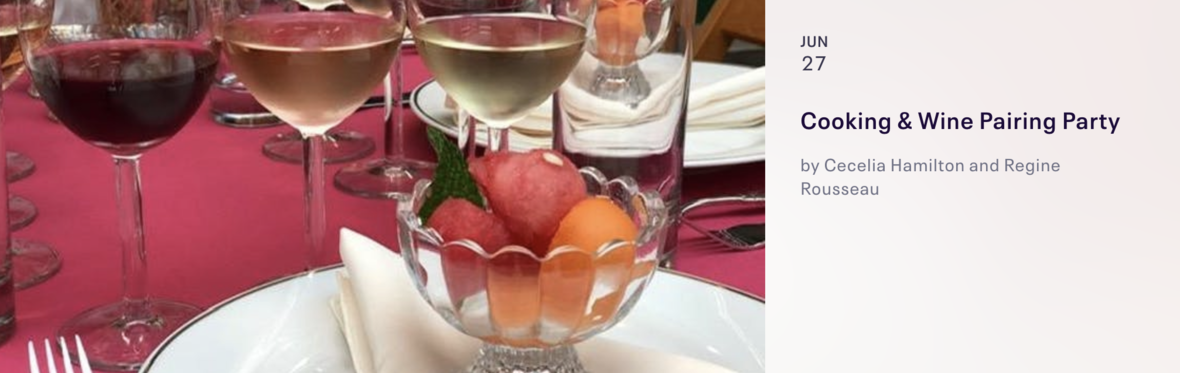/* styles */ 6/27 Cooking & Wine Pairing Party with Heartfelt Catering Chicago Join us again with a Night Out on the Town for a Cooking and Wine Pairing Party! Learn to cook the Heartfelt way and select the best wines that pair with your meal! 6:00 PM to 9:30 PM Heartfelt Catering 1640 W Hubbard St Chicago, IL 60622

# Register by 5PM on 6/21 so we can appropriately plan for your wonderful experience!

 table div table+table+table+table+table+table+table+table+table+table+table+table+table+table+table+table+table+table+table+table+table+table+table+table+table+table+table+table+table+table+table+table+table+table+table+table+table+table+table+table+table div table{width:100%;padding:0}table div table+table+table+table+table+table+table+table+table+table+table+table+table+table+table+table+table+table+table+table+table+table+table+table+table+table+table+table+table+table+table+table+table+table+table+table+table+table+table+table+table div table img{width:96.23%;padding:0;float:none}table div table+table+table+table+table+table+table+table+table+table+table+table+table+table+table+table+table+table+table+table+table+table+table+table+table+table+table+table+table+table+table+table+table+table+table+table+table+table+table+table+table div table td{width:100%;padding:0 1.88% 18px}/* styles *//* styles */ Here's an example of the cooking vibe we had during our special Mother's Day Event! Note: Each event is specially curated. Offerings will be new and differ from one another.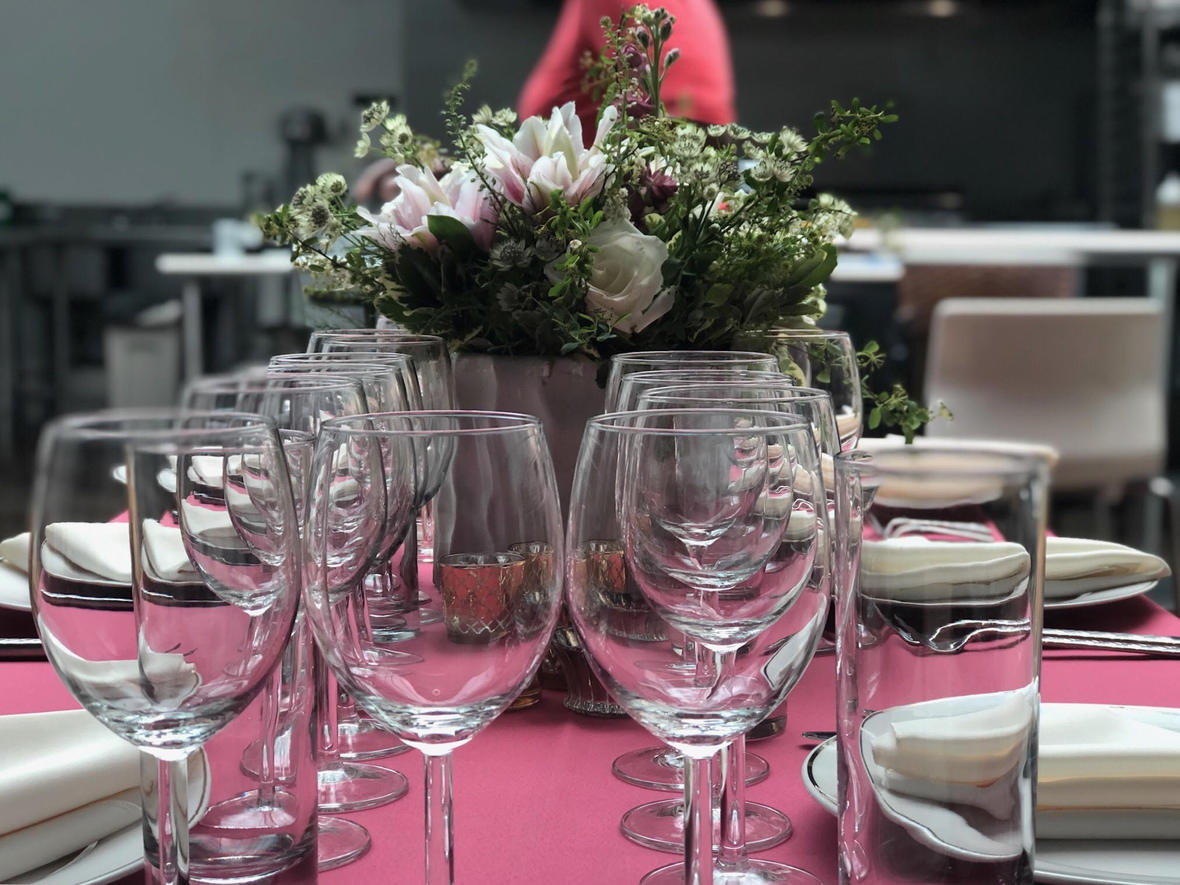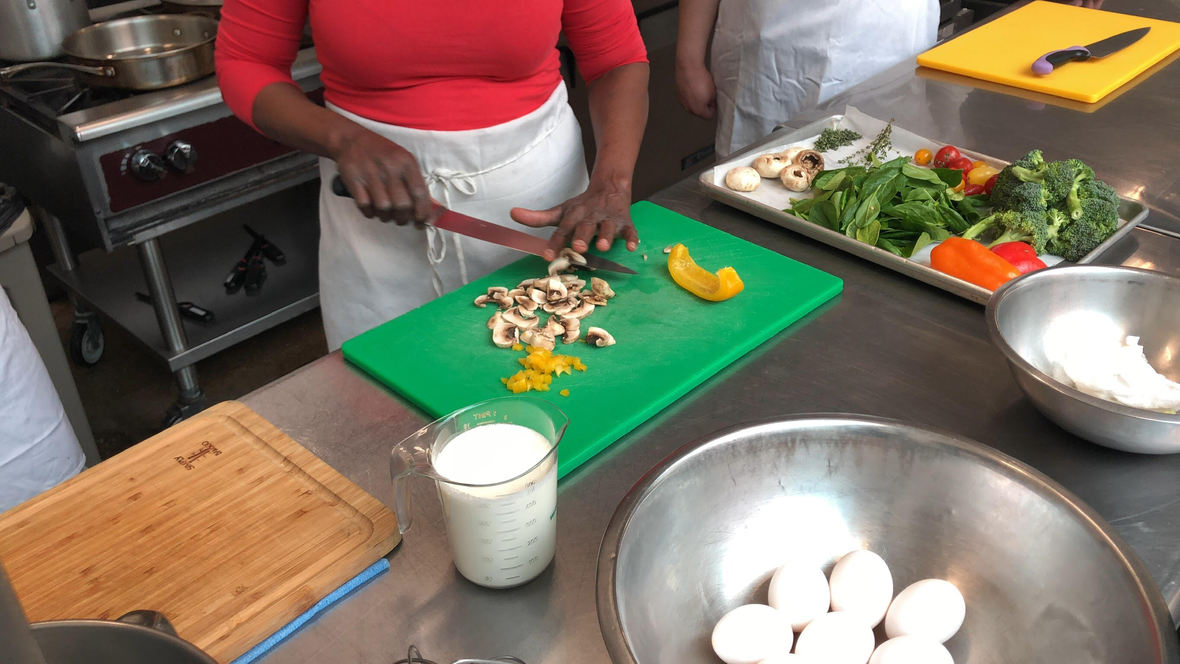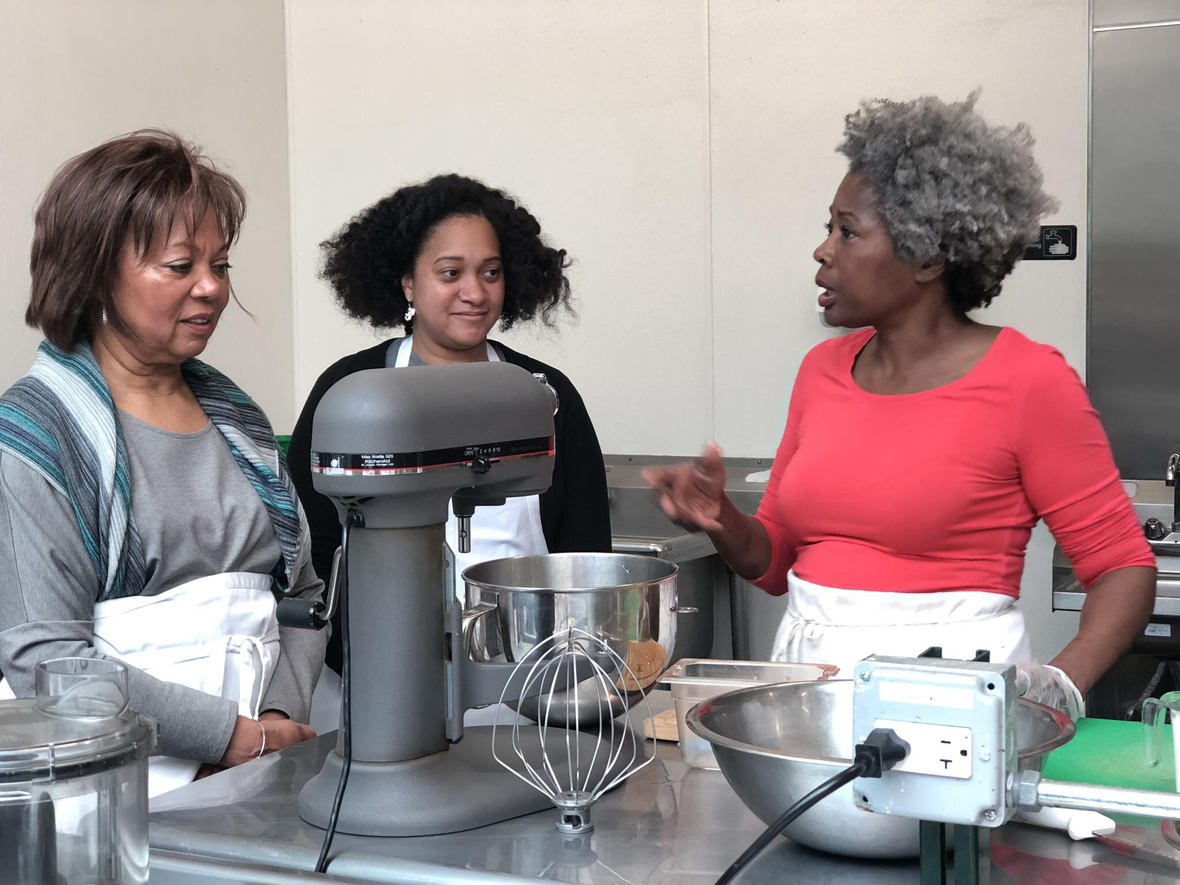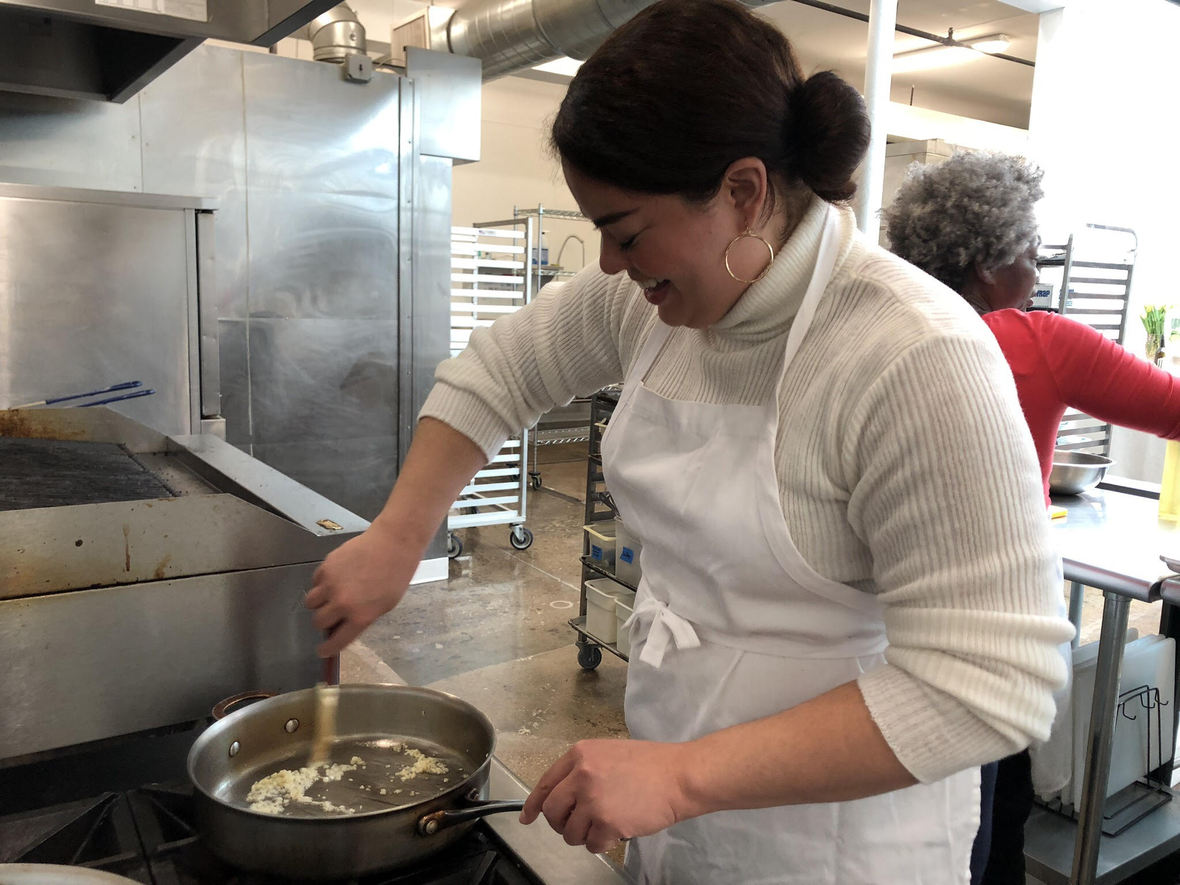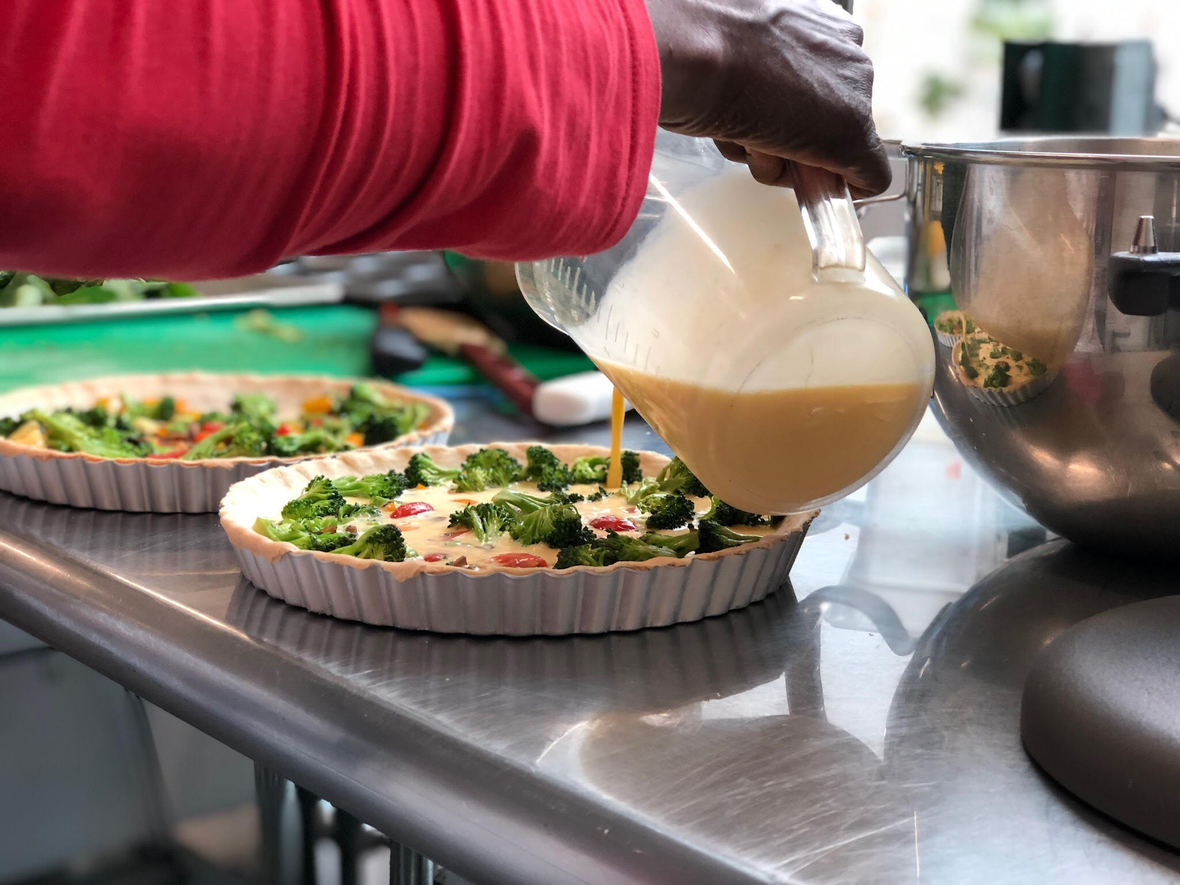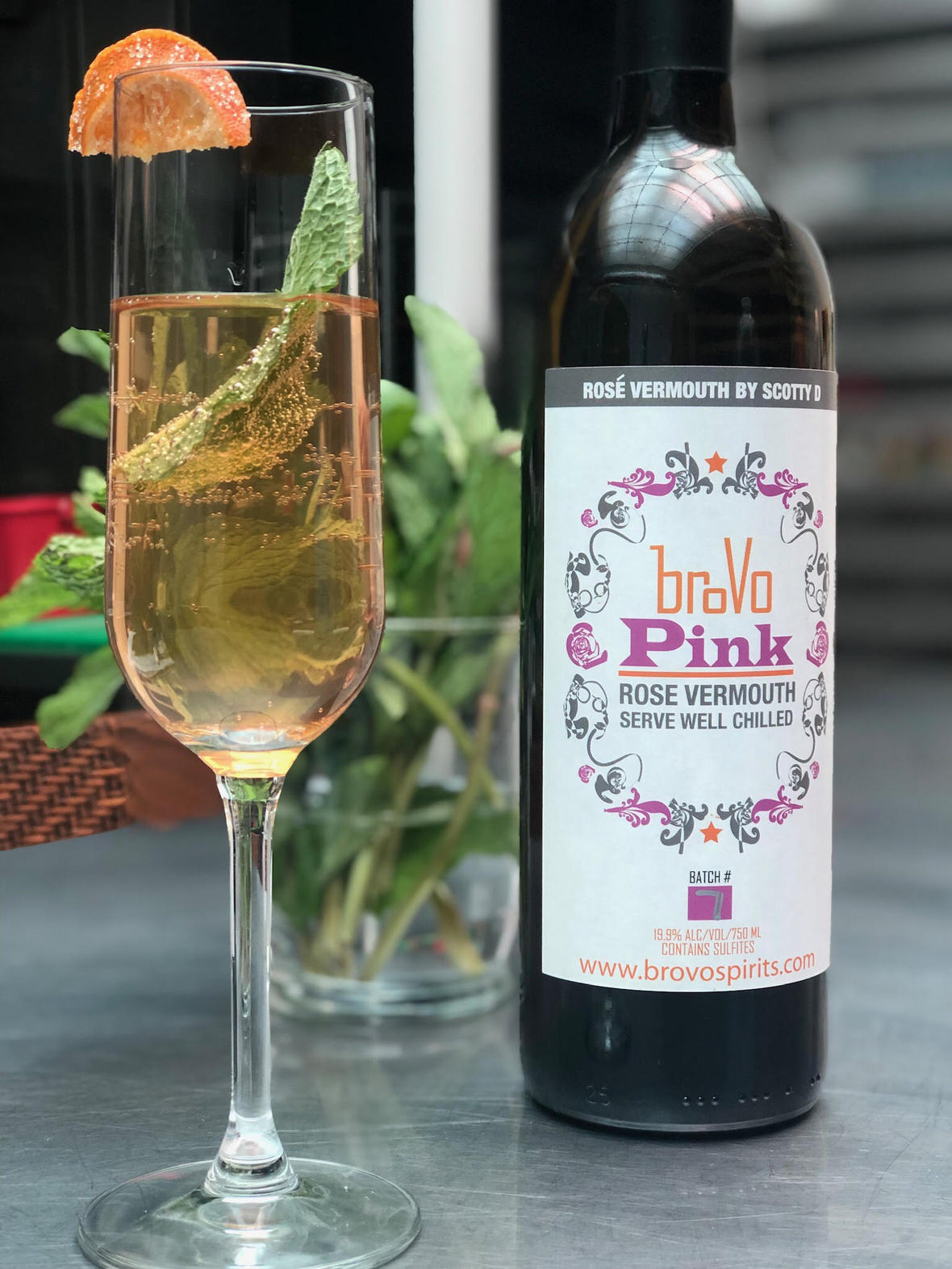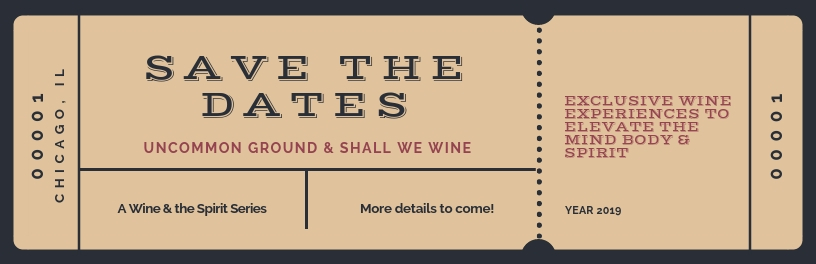# MORE SAVE THE DATES

Wine & the Spirit Series - As you know, Shall We Wine has teamed up with Uncommon Ground for a series of events this year. If you missed out on any other event, there are more chances for you to participate. More details to come!

Wednesday, August 14th - Food and Wine Cocktails
Our friends from Coquetel Spirits will partner with us to create unique wine inspired cocktails, paired with a delicious three-course meal.

Saturday, October 19th - Bubbles & Birds
Featured sparkling wine pairings for the fried goodness that is fried chicken!

Sunday, November 10th - Veterans of Music
Veterans Day weekend we honor Chicago Veterans of Music with a music & wine pairing event.

 /* styles */

# Use the hashtag #shallwewineputmeon on your posts and share!

 /* styles */table div table+table+table+table+table+table+table+table+table+table+table+table+table+table+table+table+table+table+table+table+table+table+table+table+table+table+table+table+table+table+table+table+table+table+table+table+table+table+table+table+table+table+table+table+table+table+table+table+table+table+table+table+table div table{width:100%;padding:0}table div table+table+table+table+table+table+table+table+table+table+table+table+table+table+table+table+table+table+table+table+table+table+table+table+table+table+table+table+table+table+table+table+table+table+table+table+table+table+table+table+table+table+table+table+table+table+table+table+table+table+table+table+table div table img{width:96.23%;padding:0;float:none}table div table+table+table+table+table+table+table+table+table+table+table+table+table+table+table+table+table+table+table+table+table+table+table+table+table+table+table+table+table+table+table+table+table+table+table+table+table+table+table+table+table+table+table+table+table+table+table+table+table+table+table+table+table div table td{width:100%;padding:0 1.88% 18px}/* styles */## DRINK THIS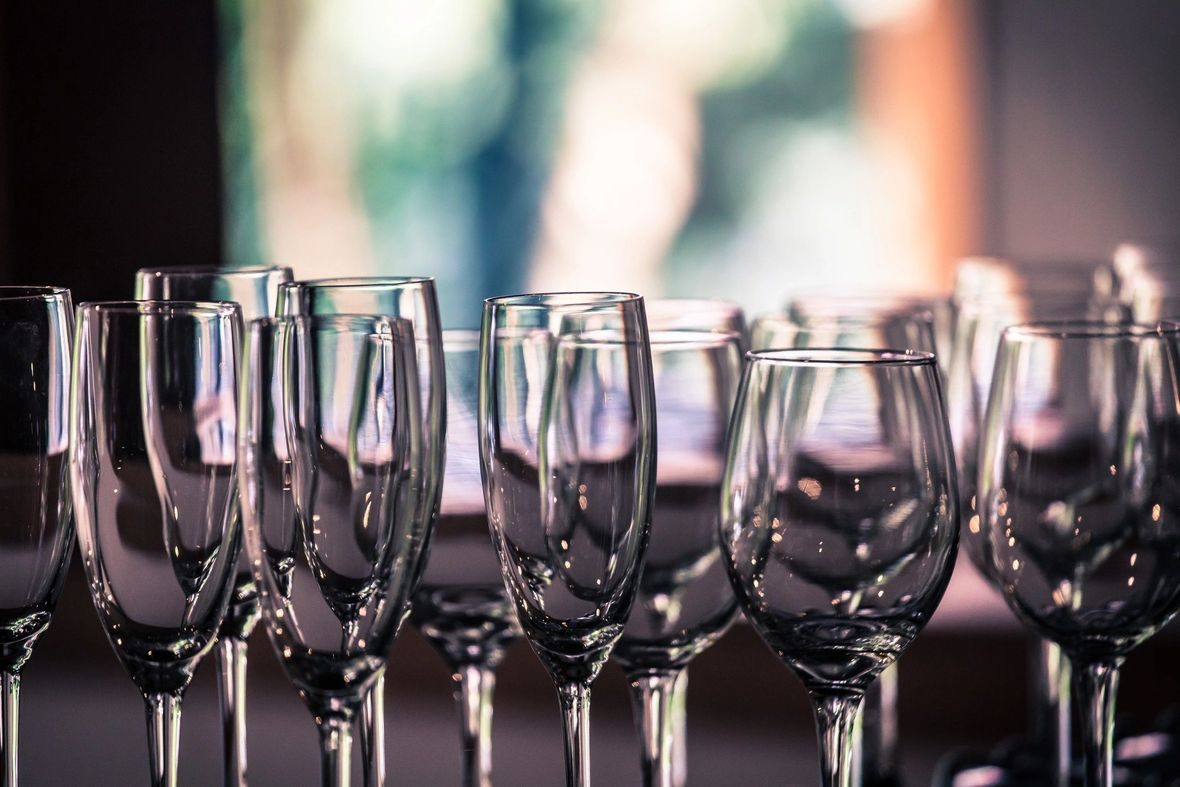# This month's wine features! Cheers!# Words & Wine - CW Chicago

 table div table+table+table+table+table+table+table+table+table+table+table+table+table+table+table+table+table+table+table+table+table+table+table+table+table+table+table+table+table+table+table+table+table+table+table+table+table+table+table+table+table+table+table+table+table+table+table+table+table+table+table+table+table+table+table+table+table+table+table div table{width:100%;padding:0}table div table+table+table+table+table+table+table+table+table+table+table+table+table+table+table+table+table+table+table+table+table+table+table+table+table+table+table+table+table+table+table+table+table+table+table+table+table+table+table+table+table+table+table+table+table+table+table+table+table+table+table+table+table+table+table+table+table+table+table div table img{width:96.23%;padding:0;float:none}table div table+table+table+table+table+table+table+table+table+table+table+table+table+table+table+table+table+table+table+table+table+table+table+table+table+table+table+table+table+table+table+table+table+table+table+table+table+table+table+table+table+table+table+table+table+table+table+table+table+table+table+table+table+table+table+table+table+table+table div table td{width:100%;padding:0 1.88% 18px}/* styles */## Q&A: TO GUZZLE OR NOT GUZZLE

 table div table+table+table+table+table+table+table+table+table+table+table+table+table+table+table+table+table+table+table+table+table+table+table+table+table+table+table+table+table+table+table+table+table+table+table+table+table+table+table+table+table+table+table+table+table+table+table+table+table+table+table+table+table+table+table+table+table+table+table+table+table div table,table.module-60{width:47.74%;float:right;padding:0}table div table+table+table+table+table+table+table+table+table+table+table+table+table+table+table+table+table+table+table+table+table+table+table+table+table+table+table+table+table+table+table+table+table+table+table+table+table+table+table+table+table+table+table+table+table+table+table+table+table+table+table+table+table+table+table+table+table+table+table+table+table div table a{border:0 none;text-decoration:none}table div table+table+table+table+table+table+table+table+table+table+table+table+table+table+table+table+table+table+table+table+table+table+table+table+table+table+table+table+table+table+table+table+table+table+table+table+table+table+table+table+table+table+table+table+table+table+table+table+table+table+table+table+table+table+table+table+table+table+table+table+table div table img{width:100%!important;border:0 none;text-decoration:none}table div table+table+table+table+table+table+table+table+table+table+table+table+table+table+table+table+table+table+table+table+table+table+table+table+table+table+table+table+table+table+table+table+table+table+table+table+table+table+table+table+table+table+table+table+table+table+table+table+table+table+table+table+table+table+table+table+table+table+table+table+table div table td{width:100%;padding:0 0 20px 20px}/* styles */ National Wine Day is near! Are you saving for something special? Will you wing it? No idea? Whatever the case, spend time with what you drink. Taking your time does not make you pretentious. Think of it like taking the time to appreciate your beverage in the same way you might with a good book, sports series, TV show or an album. Click on the image to the right to watch a video on this topic. Happy drinking!
 table div table+table+table+table+table+table+table+table+table+table+table+table+table+table+table+table+table+table+table+table+table+table+table+table+table+table+table+table+table+table+table+table+table+table+table+table+table+table+table+table+table+table+table+table+table+table+table+table+table+table+table+table+table+table+table+table+table+table+table+table+table+table div table{width:100%;padding:0}table div table+table+table+table+table+table+table+table+table+table+table+table+table+table+table+table+table+table+table+table+table+table+table+table+table+table+table+table+table+table+table+table+table+table+table+table+table+table+table+table+table+table+table+table+table+table+table+table+table+table+table+table+table+table+table+table+table+table+table+table+table+table div table img{width:96.23%;padding:0;float:none}table div table+table+table+table+table+table+table+table+table+table+table+table+table+table+table+table+table+table+table+table+table+table+table+table+table+table+table+table+table+table+table+table+table+table+table+table+table+table+table+table+table+table+table+table+table+table+table+table+table+table+table+table+table+table+table+table+table+table+table+table+table+table div table td{width:100%;padding:0 1.88% 18px}/* styles */## DID YOU KNOW...

 table div table+table+table+table+table+table+table+table+table+table+table+table+table+table+table+table+table+table+table+table+table+table+table+table+table+table+table+table+table+table+table+table+table+table+table+table+table+table+table+table+table+table+table+table+table+table+table+table+table+table+table+table+table+table+table+table+table+table+table+table+table+table+table+table div table,table.module-63{width:32.08%;float:right;padding:0}table div table+table+table+table+table+table+table+table+table+table+table+table+table+table+table+table+table+table+table+table+table+table+table+table+table+table+table+table+table+table+table+table+table+table+table+table+table+table+table+table+table+table+table+table+table+table+table+table+table+table+table+table+table+table+table+table+table+table+table+table+table+table+table+table div table a{border:0 none;text-decoration:none}table div table+table+table+table+table+table+table+table+table+table+table+table+table+table+table+table+table+table+table+table+table+table+table+table+table+table+table+table+table+table+table+table+table+table+table+table+table+table+table+table+table+table+table+table+table+table+table+table+table+table+table+table+table+table+table+table+table+table+table+table+table+table+table+table div table img{width:100%!important;border:0 none;text-decoration:none}table div table+table+table+table+table+table+table+table+table+table+table+table+table+table+table+table+table+table+table+table+table+table+table+table+table+table+table+table+table+table+table+table+table+table+table+table+table+table+table+table+table+table+table+table+table+table+table+table+table+table+table+table+table+table+table+table+table+table+table+table+table+table+table+table div table td{width:100%;padding:0 0 20px 20px}/* styles */ WHERE TO FIND SHALL WE WINE So you know us for our pop up events, but did you know that our consultants host mini pop-ups every weekend at your local wine shop and/or grocery stores? Next time you're tasting wine at Binny's, Whole Foods, Mariano's or your local wine shop, ask the consultant if they are with Shall We Wine! Sounds like a fun gig? We are hiring wine and spirit enthusiasts! Send your resume to hr@shallwewine.com and follow the link below to apply./* styles */ Learn, Drink, Repeat! The Shall We Wine Team
 Like   Tweet   Pin   +1   in